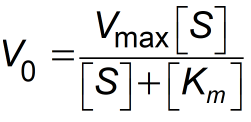Request a Tool

Michaelis Menten Equation Calculator

Michaelis-Menten equation calculator allows you to discover the kinetics of one-substrate reaction

Output

Reactive rate (V)
0

Formula• Km = Michaelis constant
• Vmax = Max reaction speed
• [S] = Substrate Concentration

Defination / Uses

The Michaelis-Menten equation calculator may be used to figure out the kinetics of a one-substrate enzyme-catalyzed reaction. The rate of the reaction, V, the substrate concentration, Km, and the maximum rate of reaction, Vmax, may all be calculated using Michaelis-Menten kinetics.

Uses

In the same way that the Langmuir equation may be used to represent generic adsorption of biomolecular species, it can be used to characterise a general biochemical process.

Enzymes' principal function is to increase reaction speeds such that they are consistent with the organism's needs. We need a kinetic description of enzyme activity to understand how they work and how the rate of catalysis V0, which is defined as the number of moles of product produced per second for various enzymes, changes with substrate concentration [S]. As the substrate concentration grows, the rate of catalysis climbs linearly, then begins to level off and approach a maximum at higher substrate concentrations. Use the upper given formula for manual calculations. No sign-up, registration OR captcha is required to use this tool.